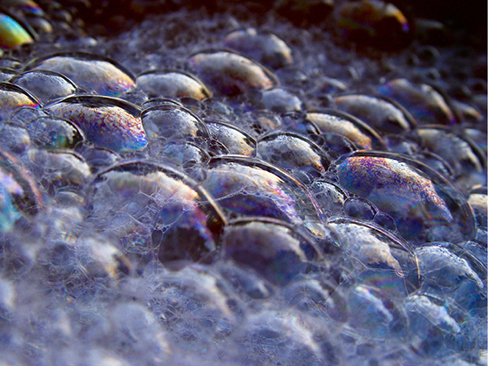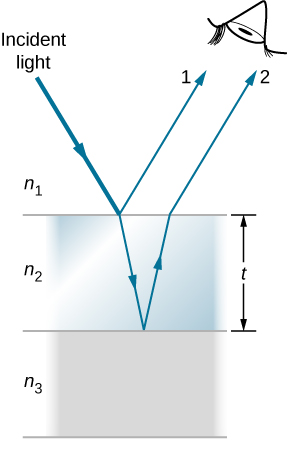# 3.4 Interference in thin films

 Page 1 / 7
By the end of this section, you will be able to:
• Describe the phase changes that occur upon reflection
• Describe fringes established by reflected rays of a common source
• Explain the appearance of colors in thin films

The bright colors seen in an oil slick floating on water or in a sunlit soap bubble are caused by interference. The brightest colors are those that interfere constructively. This interference is between light reflected from different surfaces of a thin film; thus, the effect is known as thin-film interference    .

As we noted before, interference effects are most prominent when light interacts with something having a size similar to its wavelength. A thin film is one having a thickness t smaller than a few times the wavelength of light, $\lambda$ . Since color is associated indirectly with $\lambda$ and because all interference depends in some way on the ratio of $\lambda$ to the size of the object involved, we should expect to see different colors for different thicknesses of a film, as in [link] .These soap bubbles exhibit brilliant colors when exposed to sunlight. (credit: Scott Robinson)

What causes thin-film interference? [link] shows how light reflected from the top and bottom surfaces of a film can interfere. Incident light is only partially reflected from the top surface of the film (ray 1). The remainder enters the film and is itself partially reflected from the bottom surface. Part of the light reflected from the bottom surface can emerge from the top of the film (ray 2) and interfere with light reflected from the top (ray 1). The ray that enters the film travels a greater distance, so it may be in or out of phase with the ray reflected from the top. However, consider for a moment, again, the bubbles in [link] . The bubbles are darkest where they are thinnest. Furthermore, if you observe a soap bubble carefully, you will note it gets dark at the point where it breaks. For very thin films, the difference in path lengths of rays 1 and 2 in [link] is negligible, so why should they interfere destructively and not constructively? The answer is that a phase change can occur upon reflection, as discussed next.Light striking a thin film is partially reflected (ray 1) and partially refracted at the top surface. The refracted ray is partially reflected at the bottom surface and emerges as ray 2. These rays interfere in a way that depends on the thickness of the film and the indices of refraction of the various media.

## Changes in phase due to reflection

We saw earlier ( Waves ) that reflection of mechanical waves can involve a $180\text{°}$ phase change. For example, a traveling wave on a string is inverted (i.e., a $180\text{°}$ phase change) upon reflection at a boundary to which a heavier string is tied. However, if the second string is lighter (or more precisely, of a lower linear density), no inversion occurs. Light waves produce the same effect, but the deciding parameter for light is the index of refraction. Light waves undergo a $180\text{°}$ or $\pi$ radians phase change upon reflection at an interface beyond which is a medium of higher index of refraction. No phase change takes place when reflecting from a medium of lower refractive index ( [link] ). Because of the periodic nature of waves, this phase change or inversion is equivalent to $\text{±}\lambda \text{/}2$ in distance travelled, or path length. Both the path length and refractive indices are important factors in thin-film interference.

what is bohrs model for hydrogen atom
hi
Tr
Hello
Youte
Hi
Nwangwu-ike
hi
Siddiquee
what is the value of speed of light
1.79×10_¹⁹ km per hour
Swagatika
what r dwarf planet
what is energy
কাজের একক কী
Jasim
কাজের একক কী
Jasim
friction ka direction Kaise pata karte hai
friction is always in the opposite of the direction of moving object
Punia
A twin paradox in the special theory of relativity arises due to.....? a) asymmetric of time only b) symmetric of time only c) only time
b) symmetric of time only
Swagatika
fundamental note of a vibrating string
every matter made up of particles and particles are also subdivided which are themselves subdivided and so on ,and the basic and smallest smallest smallest division is energy which vibrates to become particles and thats why particles have wave nature
Alvin
what are matter waves? Give some examples
according to de Broglie any matter particles by attaining the higher velocity as compared to light'ill show the wave nature and equation of wave will applicable on it but in practical life people see it is impossible however it is practicaly true and possible while looking at the earth matter at far
Manikant
a centeral part of theory of quantum mechanics example:just like a beam of light or a water wave
Swagatika
Mathematical expression of principle of relativity
given that the velocity v of wave depends on the tension f in the spring, it's length 'I' and it's mass 'm'. derive using dimension the equation of the wave
What is the importance of de-broglie's wavelength?
he related wave to matter
Zahid
at subatomic level wave and matter are associated. this refering to mass energy equivalence
Zahid
it is key of quantum
Manikant
how those weight effect a stable motion at equilibrium
how do I differentiate this equation- A sinwt with respect to t
just use the chain rule : let u =wt , the dy/dt = dy/du × du/dt : wA × cos(wt)
Jerry
I see my message got garbled , anyway use the chain rule with u= wt , etc...
Jerry
de broglie wave equation
vy beautiful equation
chandrasekhar
what is electro statics
when you consider systems consisting of fixed charges
SherlyBy Brooke DelaneyBy OpenStaxBy OpenStaxBy Sam LuongBy MldelatteBy Danielle StephensBy Stephen VoronBy OpenStaxBy Brooke DelaneyBy OpenStax